Empirical formula for iron oxide

Equipment

- electronic balance
- steel wool
- 9 volt battery
- butane gas lighter.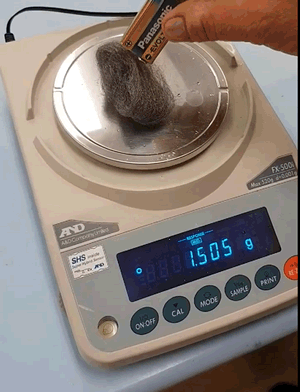If the battery is flat and will not ignite the steel then a propane gas lighter can be used. In fact, a propane gas lighter is more efficient in burning the entire steel sample.

Step 1 Weigh approximately 1.500 grams of steel wool.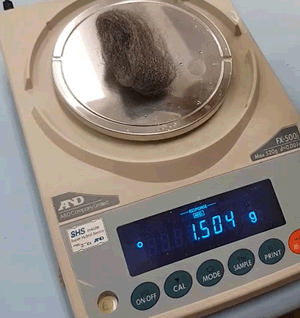Step 2 Ignite the steel by touching the electrodes of a 9-volt battery to the steel wool.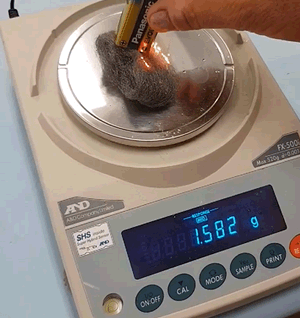Step 3 Allow the wool to slowly burn. Reignite with the battery or propane lighter if necessary.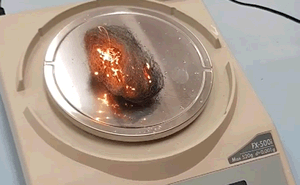Step 4 When the reaction is complete read the mass from the scale.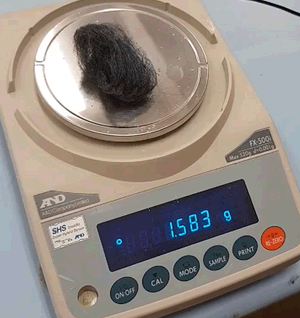1) Calculate the mol of iron present initially.

2) Calculate the mass of oxygen that reacted.

3) Calculate the mol of oxygen that reacted.

4) Find the empirical formula of the iron oxide formed.

5) What two assumptions are made in calculating the empirical formula.

Solution

6) How can you increase the reliability of your data?

7) How can you compare the accuracy of the your results?Definitions and examples of validity, accuracy and reliability are given below.

Validity relates to the experimental method. A method is valid if:
• it actually tests the hypothesis with an appropriate range of values of the independent variable.
• suitable equipment is used (e.g. a pipette is used to measure an accurate volume rather than a beaker)
• variables, other than the dependent and independent, are controlled
• assumptions are satisfied

Reliability relates to the ability of the experiment when repeated to derive similar results . An experiment is reliable if it can be repeated obtained to obtain consistent results within an acceptable range. Reliability is improved if the experiment is repeated many times and the results averaged. Repetition minimises random errors and identifies outliers which can be discarded. A discussion on reliability should include mention of any random errors that have been identified. Random errors reduce reliability.

Accuracy is dependent on reliability and validity. It is indicative of how close the results are to the true value of what is being measured. Accurate results can be substantiated in the literature or secondary studies. Accuracy is improved through the experimental method, such as, using the right equipment to take measurements and implementing ways to reduce systematic error. Use the space on the reverse side if needed. Systematic errors reduce accuracy.

Print a PDF of this investigation.